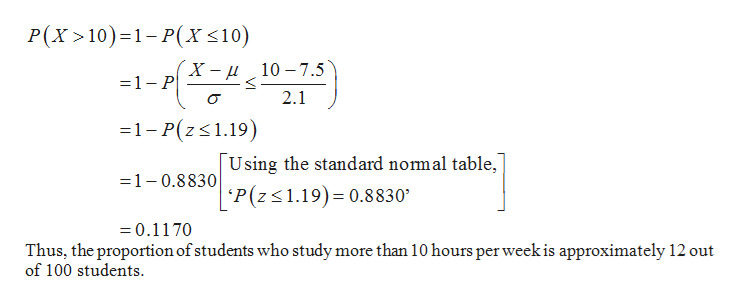# The amount of time devoted to studying statistics each week by students who achieve a grade of A in the course is a normally distributed random variable, with a mean of 7.5 hours and a standard deviation of 2.1 hours. Also indicate whether the event is usual or unusual A.  What proportion of A students study more than 10 hours per week B.  Find the probability that an A student spends between 7 and 9 hours studying C.  What proportion of A students spend fewer than 3 hours studying *Please do not solve using Microsoft Excel.  My professor is not teaching using excel.  I would like to see the steps involved to solving the problem for my own study reference for exams.*

Question

The amount of time devoted to studying statistics each week by students who achieve a grade of A in the course is a normally distributed random variable, with a mean of 7.5 hours and a standard deviation of 2.1 hours. Also indicate whether the event is usual or unusual

A.  What proportion of A students study more than 10 hours per week

B.  Find the probability that an A student spends between 7 and 9 hours studying

C.  What proportion of A students spend fewer than 3 hours studying

*Please do not solve using Microsoft Excel.  My professor is not teaching using excel.  I would like to see the steps involved to solving the problem for my own study reference for exams.*

check_circle

Step 1

Introduction:

Consider X as a normally distributed random variable, with mean μ and standard deviation σ. Then, the z-score for a particular value X = x, is:

z = (x μ)/σ.

Step 2

a.Finding the proportion of students who study more than 10 hours per week:

Here, the distribution of amount of time devoted for study follows a normal distribution with mean 7.5 hours and standard deviation 2.1 hours.

Then, the proportion of students who study more than 10 hours per week is obtained as follows:help_outlineImage TranscriptioncloseP(X 10) 1-P(x <10) 1-H*z2,012) X-u10 -7.5 2.1 -1-P(zs1.19) Using the standard nomal table, 'P(z 1.19) 0.8830 =1-0.8830 =0.1170 Thus, the proportion of students who study more than 10 hours per week is approximately 12 out of 100 students fullscreen
Step 3

b.Computing the probability that a student spends between 7 and 9 hours studying:

The probability tha...

### Want to see the full answer?

See Solution

#### Want to see this answer and more?

Solutions are written by subject experts who are available 24/7. Questions are typically answered within 1 hour.*

See Solution
*Response times may vary by subject and question.
Tagged in

### Statistics